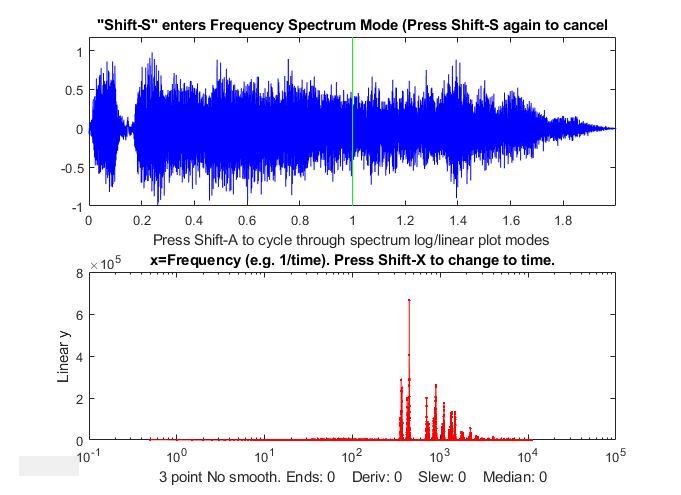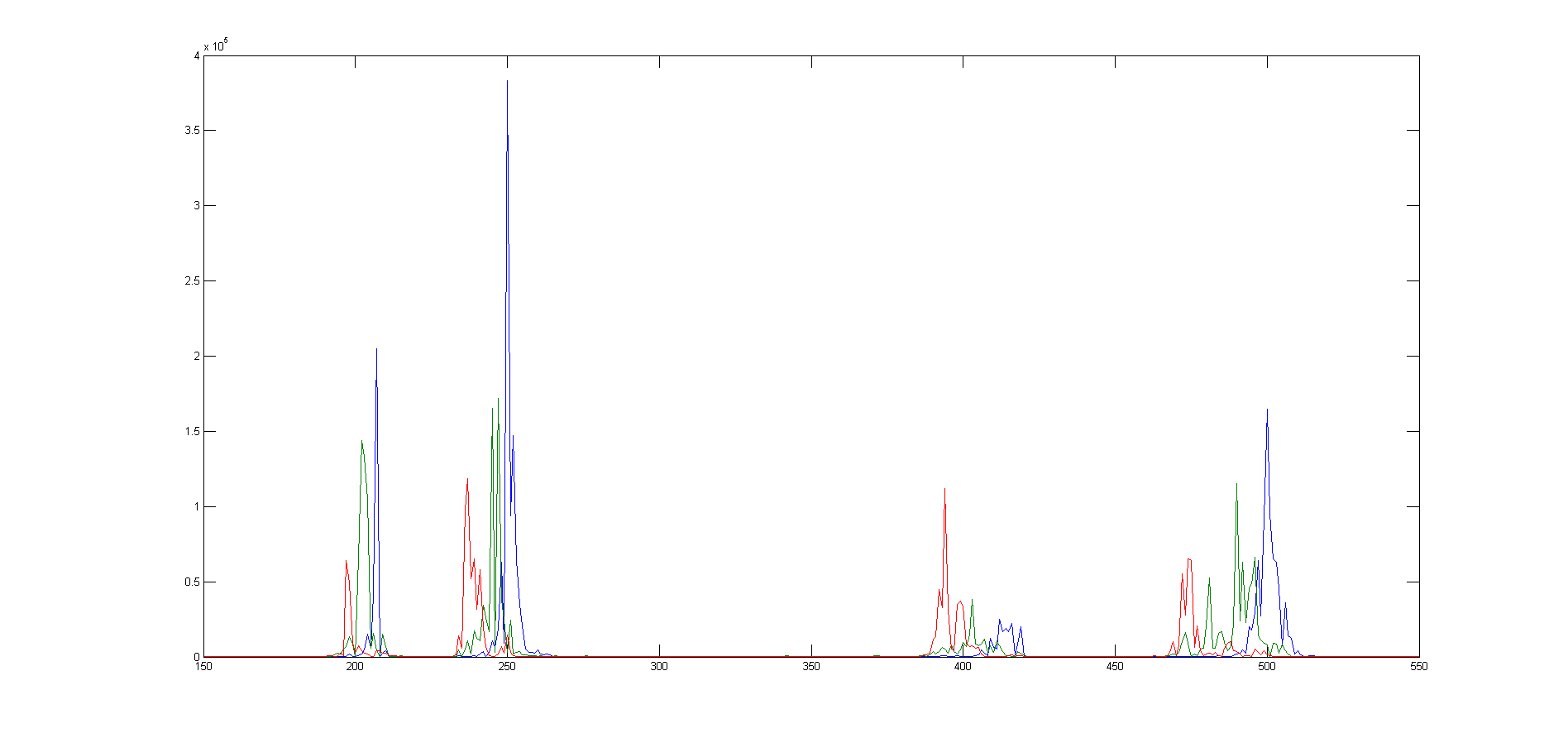[Introduction]  [Signal arithmetic]  [Signals and noise]   [Smoothing]   [Differentiation]  [Peak Sharpening]  [Harmonic analysis]   [Fourier convolution]  [Fourier deconvolution]  [Fourier filter]  [Wavelets]   [Peak area measurement]  [Linear Least Squares]  [Multicomponent Spectroscopy]  [Iterative Curve Fitting]  [Hyperlinear quantitative absorption spectrophotometry] [Appendix and Case Studies]  [Peak Finding and Measurement]  [iPeak]   [iSignal]  [Peak Fitters]   [iFilter]  [iPower]  [List of downloadable software]  [Interactive tools]

### Appendix F: Harmonic Analysis of the Doppler Effect

The wav file "horngoby.wav" (Ctrl-click to open) is a 2-second recording of the sound of a passing automobile horn, exhibiting the familiar Doppler effect. The sampling rate is 22000 Hz. Downloadthis file and place it in your Matlab path. You can then load this into the Matlab workspace as the variable "doppler" and display it using iSignal:

t=0:1/21920:2;
isignal(t,doppler);

Within iSignal, you can switch to the frequency spectrum mode (press Shift-S), and zoom in on different portions of the waveform, so you can observe the downward frequency shift and measure it quantitatively. (Actually, it's much easier to hear the frequency shift - press Shift-P to play the sound - than to see it graphically; the shift is rather small on a percentage basis, but human hearing is very sensitive to small pitch (frequency) changes). It helps to re-plot the data to stretch out the frequency region around the fundamental frequency or one of the harmonics. I used iSignal to zoom in on three slices of this waveform and then I plotted the frequency spectrum (Shift-S) near the beginning (plotted in blue), middle (green), and end (red) of the sound. The frequency region between 150 Hz and 550 Hz are plotted in the figure below:The group of peaks near 200 are the fundamental frequency of the lowest note of the horn and the group of peaks near 400 are its second harmonic. (Pitched sounds usually have a harmonic structure of 1, 2, 3... times a fundamental frequency). The group of peaks near 250 are the fundamental frequency of the next higher note of the horn and the group of peaks near 500 are its second harmonic. (Car and train horns often have two or three harmonious notes sounded together). In each of these groups of harmonics, you can clearly see that the blue peak (the spectrum measured at the beginning of the sound) has a higher frequency than the red peak (the spectrum measured at the end of the sound). The green peak, taken in the middle, has an intermediate frequency. The peaks are ragged because the amplitude and frequency varies over the sampling interval, but you can still get good quantitative measures of the frequency of each component by curve fitting to a Gaussian peak model using peakfit.m or ipf.m:

Peak   Position  Height      Width    Area
Start     206.69  3.02e+005   0.8187  2.46e+005
Middle  202.65  1.55e+005   2.911    4.8e+005
End      197.42   81906          1.378   1.2e+005

The estimated precision of the peak position (i.e. frequency) measurements is about 0.2% relative, based on the bootstrap method, good enough to allow accurate calculation of the frequency shift (about 4.2%) and to demonstrate that the ratio of the second harmonic to the fundamental for these data is 2.0023, which is very close to the theoretical value of 2. You could even calculate the the speed of the vehicle.

It's also possible to plot the evolving spectrum of such a signal as a contour graph, using the PlotSegFreqSpect.m function, as show in the graphic below. You can see the various frequency components drifting steadily down in frequency as the time passes. See HarmonicAnalysis.html#Software.

PSM=PlotSegFreqSpect(t,doppler,40,350,0); % 40 segments, 240 harmonics

This page is part of "A Pragmatic Introduction to Signal Processing", created and maintained by Tom O'Haver, Professor Emeritus, Department of Chemistry and Biochemistry, The University of Maryland at College Park. Comments, suggestions and questions should be directed to Prof. O'Haver at toh@umd.edu. Updated July, 2022.